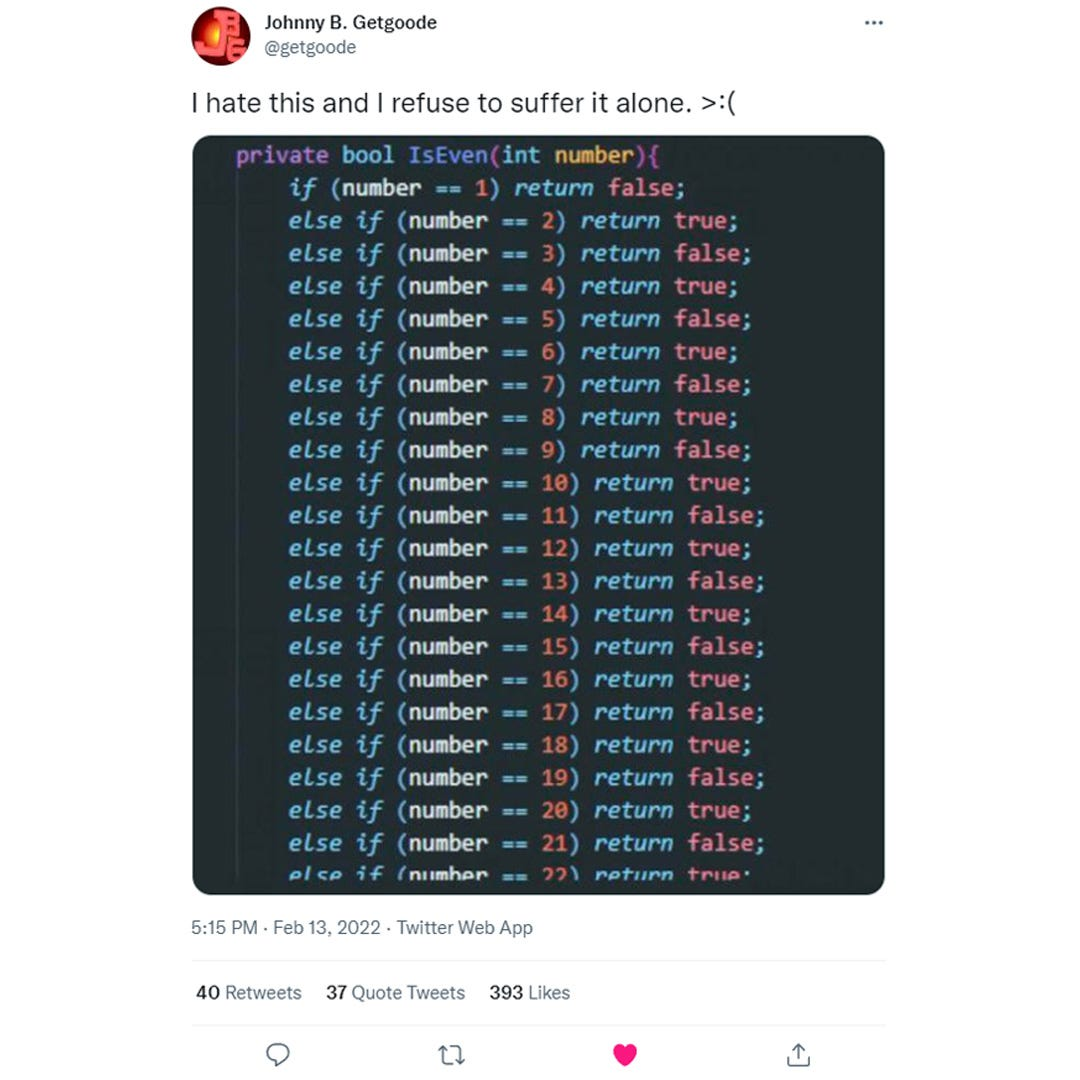# Un-Cringing An is_even() Function in PythonJohnny B. Getgoode tweeted this screenshot of if-else statements.

Johnny B. Getgoode tweeted a screenshot from his developer Discourse server on February 13, 2022. Experienced programmers cringed at the repeating lines of if else to determine if a number is even or odd. That prompted a lively discussion on how to “un-cringe” this function.

Here are four ways to refactor the function for readability and speed in Python: match and case, recursive inner function, modulo operator, and bitwise operator.

## Original Function in Python

I rewrote the function in Python using elif as else if would cause a syntax error. For the sake of brevity, I limited the defined numbers to ten. Even numbers return True, odd numbers return False. For undefined numbers, the catch-all else returns False.

Although I’m using Python as my programming language, the refactoring examples can apply to any programming language.

## 1. Match & Case

We can refactor the function to use match and case as a replacement for elif. Python had lacked the traditional switch and case statements found in other programming languages for a great many years. That changed with structural pattern matching in Python 3.10, adding match and case as the functional equivalent of switch and case.

Using match and case makes the function more readable and faster than elif. Each number must be defined, and the catch-all case (“_”) return False for undefined numbers.

## 2. Recursive Inner Function

We can refactor the function to use a recursive inner function that calls itself to subtract 2 from the starting number until the number is less than 2, and then use % (modulo operator) with 2 and compare the remainder with zero to return a Boolean. Even numbers are divisible by 2 and don’t have a remainder.

Unlike elif or match and case, the recursive inner function can test any number without having to define it first. While shorter than the previous two functions, this function can be refactored as a one liner (see line 4).

## 3. Modulo Operator

We can refactor the function to use % (modulo operator) with 2 and compare the remainder with zero to return a Boolean. Even numbers are divisible by 2 and don’t have a remainder.

Most programmers would stop here. The modulo operator is commonly used for determining even or odd numbers. But it’s not the fastest one liner.

## 4. Bitwise Operator

We can refactor the function to use & (bitwise operator) to compare the Least Significant Bit (LSB) of a number with the number 1 (0b00000001). Even numbers have 0 for the LSB (i.e., 2 = 0b00000010), odd numbers have 1 for the LSB (i.e., 3 = 0b00000011). The not operator is needed to return True for an even number and False for an odd number.

Since the bitwise operator is a binary comparison, it’s the fastest one liner for refactoring the original function.

If you enjoyed reading this essay, please follow me on Medium.

--

--